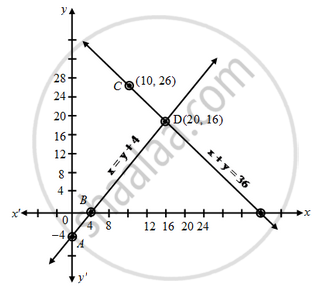Share

# Half the perimeter of a garden, whose length is 4 more than its width is 36m. Find the dimensions of the garden - CBSE Class 10 - Mathematics

ConceptGraphical Method of Solution of a Pair of Linear Equations

#### Question

Half the perimeter of a garden, whose length is 4 more than its width is 36m. Find the dimensions of the garden

#### Solution

Let the length of the garden be x and width of the garden be y.

Then the equation formed are

x = y + 4 ….(1)

Half perimeter = 36

x + y = 36 ….(2)

y = x – 4

 x 0 4 y -4 0 Points A B

y = 36 – x

 x 10 20 y 26 16 Points C D

Plotting these points we draw the lines AB and CD passing through them to represent the equationsThe two lines AB and CD intersect at the point (20, 16), So, x = 20 and y = 16 is the required solution of the pair of linear equations i.e. length of the garden is 20 m and width of the garden is 16 m.

Verification:
Putting x = 20 and y = 16 in (1). We get

20 = 16 + 4 = 20, (1) is verified.

Putting x = 20 and y = 16 in (2). we get

20 + 16 = 36

36 = 36, (2) is verified.

Hence, both the equations are satisfied

Is there an error in this question or solution?
Solution Half the perimeter of a garden, whose length is 4 more than its width is 36m. Find the dimensions of the garden Concept: Graphical Method of Solution of a Pair of Linear Equations.
S